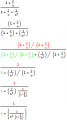# Missing a few steps for some fancy math - transistor gain

#### KevinEamon

Joined Apr 9, 2017
281

#### Jony130

Joined Feb 17, 2009
5,243
Well, from what I see to get "1" in the numerator you need to divide the numerator by ( 1 + 2/β) , or multiply by the reciprocal . Of course, the denominator also needs to be divided by ( 1 + 2/β ).
But what all of these math manipulations have anything to do with the actual electronics? Do you need to memorize this equation or what?

•KevinEamon

#### KevinEamon

Joined Apr 9, 2017
281
Yeh but what I tend to do to memorize these things, is to actually do it. Piece by piece then if I get stuck I just go through the steps.

#### KevinEamon

Joined Apr 9, 2017
281
•Zeeus

#### Jony130

Joined Feb 17, 2009
5,243
But you can simplify it further.

$\beta^{2}(1+\frac{2}{\beta} )$
$\beta^{2}+\frac{2}{\beta} \cdot \beta^2$
$\beta^{2}+\frac{2 \beta^2}{\beta}$
$\beta^{2}+\frac{2 \beta \beta}{\beta}$
$\beta^{2}+2 \beta$

But do you know how the author of a book gets the first (original) equation?

•KevinEamon

#### Zeeus

Joined Apr 17, 2019
615
•KevinEamon

#### The Electrician

Joined Oct 9, 2007
2,848
Hi guys
I'm just going through my notes here.

My lecturer has gone from this equation to the next - in a single bound!
Now I can see they are equal substituting in a value for beta -I could just learn that and great 100%
But that's not the way my brain works. I have to know the steps in between to see how it forms together.

View attachment 190589
Do it like this:Last edited:
•KevinEamon

#### KevinEamon

Joined Apr 9, 2017
281
Lol Zeeus. That's actually what the circuit is.
However, I think the question Jony was posing, was one of a more philosophical nature#### MrAl

Joined Jun 17, 2014
8,477
Hi,

Well you are given the answer so you can set up two equations and try to figure out what works for both.
One equation comes from the numerator of the first set multiplied by a constant K and the numerator of the answer set and the other equation comes from the denominator of the first set multiplied by a constant K and the denominator of the answer. We end with with these two equations:
K*(2/B+1)=1
K*(2/B+2/B^2+1)=2/((2/B+1)*B^2)+1

Now solve these two for K and get B/(B+2) which is the required factor to get the numerator and denominator of the actual answer.